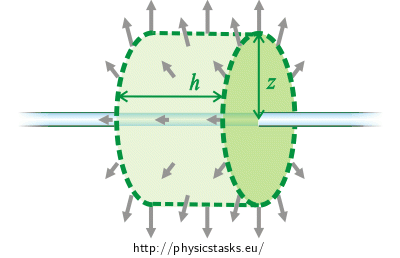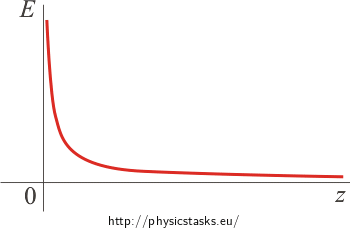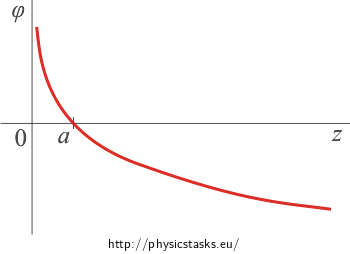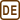## Field of Charged Line

An infinite line is uniformly charged with a linear charge density λ.

1) Find a formula describing the electric field at a distance z from the line.

2) Determine the electric potential at the distance z from the line.

• #### Hint: Electric field intensity

Giving the fact, that the line is symmetrical, we will solve this task by using Gauss's law. It is therefore necessary to choose a suitable Gaussian surface.

• #### Hint: Potential

Potential is equal to potential energy per unit charge

$\varphi\,=\, \frac{E_p}{Q}$

and potential energy is equal to negative taken work done by electric force needed to transfer a unit charge from a point of zero potential energy (in our case we choose this place to be at a distance a from the line) to a given point.

$E_p(z)\,=\, - \int^z_{a} \vec{F} \cdot \mathrm{d}\vec{z}$

Note: If we choose the point of zero potential energy to be in infinity, as we do in the majority of the tasks, we are not able to calculate the integral.

By dividing both sides of the equation by charge Q, we obtain:

$\varphi\,=\, - \int^z_{a} \frac{\vec{F}} {Q}\cdot \mathrm{d}\vec{z}\,.$

Electric force $$\vec{F}$$ divided by charge Q is equal to electric field intensity $$\vec{E}$$.

$\varphi\,=\, - \int^z_{a} \vec{E}\cdot \mathrm{d}\vec{z}$
• #### Analysis

Due to the symmetric charge distribution the simplest way to find the intensity of electric field is using Gauss's law. Gauss's law relates the electric flux in a closed surface and a total charge enclosed in this area.

The symmetry of the charge distribution implies that the direction of electric intensity vector is outward the charged line and its magnitude depends only on the distance from the line. This is due to a symmetrical distribution of the charge on the line.

We choose the Gaussian surface to be a surface of a cylinder with its axis coinciding with the line. In such a case, the vector of electric intensity is perpendicular to the lateral area of the cylinder and is of the same magnitude at all points of the lateral area. The vector is parallel to the bases of the cylinder; therefore the electric flux through the bases is zero. This simplifies the calculation of the total electric flux. Only a part of the charged line is enclosed inside the Gaussian cylinder, which means that only a corresponding part of total charge is enclosed in this surface.

We determine the electric potential using the electric field intensity. Electric potential at a given point is equal to a negative taken integral of electric intensity from the point of zero potential to the given point. We choose the point of zero potential to be at a distance z from the line. (A more detailed explanation is given in Hint.)

• #### Solution: Intensity

In this section we determine the intensity of electric field at a distance z from the charged line.

We use Gauss's law:

$\oint_S \vec{E} \cdot \mathrm{d}\vec{S}\,=\, \frac{Q}{\varepsilon_0}$ $\oint_S \vec{E} \cdot \vec{n}\mathrm{d}S\,=\, \frac{Q}{\varepsilon_0}\tag{*}$

The charge is distributed uniformly on the line, so the electric field generated by the straight line is symmetrical. The vector of electric intensity is directed radially outward the line (i.e. it is perpendicular to the line), and its magnitude depends only on the distance from the line.We choose the Gaussian surface to be a surface of a cylinder (in the figures illustrated by green), the axis of this cylinder coincides with the line. Total electric flux through this surface is obtained by summing the flux through the bases and the lateral area of the cylinder.

Flux through bases:

The vector of electric field intensity is parallel to the bases of the Gaussian cylinder; therefore the electric flux is zero. The total electric flux through the Gaussian surface is equal only to the flux through the lateral area of the Gaussian cylinder.

Flux through lateral area:

The vector of electric field intensity is perpendicular to the lateral area of the cylinder, and therefore $$\vec{E} \cdot \vec{n}\,=\,En\,=\,E$$ applies. (Note: $$\vec{n}$$ in a unit vector).

Using this knowledge, we can evaluate the electric flux through the lateral area and adjust the integral on the left side of the Gauss's law:

$\oint_{la} \vec{E} \cdot \vec{n}\mathrm{d}S\,=\,\oint_{la} E n\mathrm{d}S\,=\, \oint_{la} E\mathrm{d}S\,.$

The vector of electric field intensity $$\vec{E}$$ is at all points of the lateral area of the same magnitude; therefore it can be factored out of the integral as a constant. We obtain

$\oint_{la} \vec{E} \cdot \vec{n}\mathrm{d}S\,=\,E \oint_{la} \mathrm{d}S\,=\,E S_{la}\,,$

where Sla = 2πzl is a surface of the cylinder lateral area (l is the length of the cylinder).

$\oint_{la} \vec{E} \cdot \vec{n}\mathrm{d}S\,=\,E\, 2 \pi z l$

The resulting relation is substituted back into Gauss's law (*).

$E 2 \pi z l\,=\, \frac{Q}{\varepsilon_0}$

We evaluate the electric intensity.

$E \,=\, \frac{Q}{2 \pi \varepsilon_0 z l}\tag{**}$

Now we need to evaluate charge Q enclosed inside the Gaussian cylinder using the given values.

A part of the charged line of length l is enclosed inside the Gaussian cylinder; therefore, the charge can be expressed using its length and linear charge density λ.

$Q\,=\,\lambda l$

By substituting into the formula (**) we obtain

$E \,=\, \frac{\lambda l}{2 \pi \varepsilon_0 z l}$

After adjusting the result we obtain, that the electric field intensity of a charged line is at a distance z described as follows:

$E \,=\, \frac{ \lambda }{2 \pi \varepsilon_0\,z }\,.$

We can see that the electric intensity of a charged line decreases linearly with distance z from the line.

• #### Solution: Potential

The potential at a given point is equal to a negative taken integral of electric intensity from the point of zero potential to the given point. We select the point of zero potential to be at a distance a from the charged line. (A more detailed explanation is given in Hint.)

$\varphi (z)\,=\, - \int_{a}^z \vec{E} \cdot \mathrm{d}\vec{z}$

Note: If we select the point of zero potential to be in infinity, as we do in the majority of the tasks, we cannot calculate the integral.

The potential does not depend on the choice of the path of integration so it can be chosen at will. In this task, we choose the path of integration to be a part of a straight line perpendicular to the charged line.

The vector of electric field intensity $$\vec{E}$$ is parallel to the $$\vec{z}$$ vector. Therefore, we can simplify the integral.

$\varphi (z)\,=\, - \int^{z}_{a} E \mathrm{d}z$

We substitute the magnitude of the electric intensity vector determined in the previous section into this integral an we factor all constants out of the integral.

$\varphi (z)\,=\, - \int^{z}_{a} \frac{\lambda}{2 \pi \varepsilon_0}\,\frac{1}{z}\, \mathrm{d}z \,=\, - \frac{\lambda}{2\pi \varepsilon_0} \int^{z}_{a}\frac{1}{z}\, \mathrm{d}z\,.$

Now we calculate the integral.

$\varphi (z)\,=\,- \,\frac{\lambda}{2\pi\varepsilon_0}\left[\ln z\right]^z_{a}\,.$

We substitute the limits of the integral and factor constants out:

$\varphi (z)\,=\,-\frac{\lambda}{2\pi\varepsilon_0}\, \ln z\,+\,\frac{\lambda}{2\pi\varepsilon_0}\, \ln a\,=\,\frac{\lambda}{2\pi\varepsilon_0}\, \left(\ln a\,-\, \ln z\right)\,.$

The difference of logarithms is the logarithm of division.

$\varphi (z)\,=\,\frac{\lambda}{2\pi\varepsilon_0}\, \ln \frac{a}{z}$

We have obtained the electric potential outside the Gaussian cylinder at distance z.

The electric intensity at distance z is described as follows

$E \,=\, \frac{\lambda}{2 \pi \varepsilon_0 \,z}\,.$

The vector of electric intensity points outward the straight line (if the line is positively charged).

The electric field potential of a charged line is given by relation

$\varphi (z)\,=\,\frac{\lambda}{2\pi\varepsilon_0}\, \ln \frac{a}{z}\,.$
• #### Graphs

When drawing the graphs, we consider the line to be positively charged.

Graph of electric intensity as a function of a distance from the cylinder axis

At a distance z the vector pointing outward the line is of magnitude:v

$E \,=\, \frac{ \lambda}{ 2\pi \varepsilon_0\,z}\,.$The function is continuous. This is an inverse proportion, i.e. the graph is one branch of a hyperbola.

Note: The electric field is continuous except for points on a charged surface. When passing the charged surfaces the only thing remaining continuous is the tangent component of the intensity vector. The normal component changes "by steps" which are proportional to the surface charge density. In this task there are no charged surfaces.

Graph of electric potential as a function of a distance from the cylinder axisThe electric potential at a distance z is

$\varphi (z)\,=\,\frac{\lambda}{2\pi \varepsilon_0}\, \ln \frac{a}{z}\,.$

The function is continuous on the whole interval. It intersects the z axis at point a where we have chosen the potential to be zero.

Note: Electric potential is always continuous, because it is actually work done by transferring a unit charge and it can not be changed "by steps". First derivatives of potential are also continuous, except for derivatives at points on a charged surface. I.e. the potential is a smooth function.

• #### Link: How else can we determine the intensity in the neighbourhood of a charged line?

We can determine the electric field intensity of a charged line by direct integration.